﻿ Rolling <!--Induction_and_Inductance -->

## General Physics (calculus based) Class Notes

Dr. Rakesh Kapoor, M.Sc., Ph.D.

Former Faculty-University of Alabama at Birmingham, Birmingham, AL 35294

Rolling

In this chapter we will learn about different aspects of rolling objects.
After finishing this chapter we should know following topics:

Rolling of circular objects.

Relation between linear velocity of a rolling object and its angular velocity.

Rolling as a combination of pure Translation & Rotation.

Kinetic energy of rolling objects.

Importance of friction in rolling objects.

Computation of linear acceleration of few rolling objects.

A circular wheel and a rectangular block were let to go down (Click start) from the top (height h) of an incline. Both the objects are of same material and have same mass m. If energy loss due to friction is negligible, which one will arrive first to the ground?

What is rolling?

When an object rolls smoothly (without slipping) along a surface. The center of the object (blue point) moves in a straight line with velocity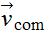while a point (red point) on the rim rotates.

Without slipping means the velocity of point of contact with horizontal surface, should always be zero.

What will be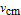(center of mass velocity) of a rolling object?

Roll (Click roll) the wheel of radius R.

Suppose the wheel covers distance s in time t, the magnitude of velocity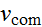of the center of mass of the wheel is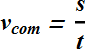When the roiling wheel rotates by an angle θ the linear distance s covered by the wheel is equal to the distance from point A to B on the wheel rim.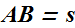According to definition of angle θ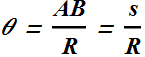Above equation can be rewritten asSince radius of the wheel R is a constant, therefore the magnitude of velocity of the center of mass of the wheel, can be rewritten as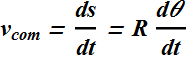For a rotating wheel, angular velocity ω is given as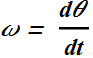Therefore velocity of the center of mass of a rolling wheel, is related to its angular velocity ω.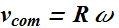Similarly the linear acceleration of the center of mass of a rolling wheel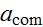is related to its angular acceleration α.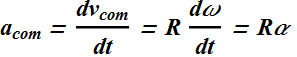The velocity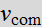and acceleration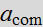of the center of mass of a smoothly rolling wheel, are respectively related to the angular velocity ω and angular acceleration α of the wheel.

What is the velocity of different points of a rolling object?

Look at the photograph of a rolling bicycle wheel.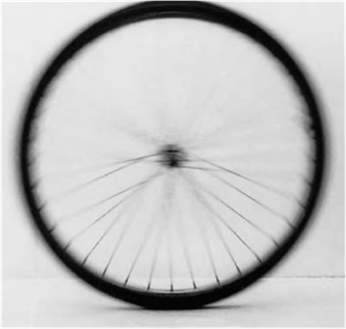What we observe?

The spokes near the wheel's top are more blurred than those near the bottom because the top ones are moving faster.

For smooth rolling (without slipping), point touching the ground should have zero velocity.

Rolling: Combination of pure Translation & Rotation

This complicated velocity pattern of a rolling object can be simplified by treating rolling as a combination of pure translation and pure rotation.

Watch a translating wheel without rotation. (Select Translation and click Play)

We observe that velocity of the wheel at point T, O and P is same.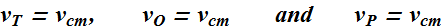Watch now a rotating wheel of radius R and angular velocity ω about a stationary axis O. (Select Rotation and click Play)

Velocity of a wheel at point T, O and P isWatch a rolling (Translation + Rotation) wheel.  (Select combine and click Play)

Now velocity of the wheel at point T, O and P is sum of two velocities due to rotation and translation.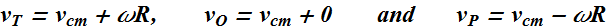For a smoothly rolling (without slipping) object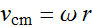, therefore velocity of the wheel at point T, O and P are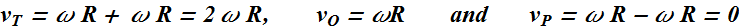Quiz- 1
Which one of the following statements concerning a wheel undergoing rolling motion is true?

The angular acceleration of the wheel must be zero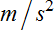.

The tangential velocity is the same for all points on the wheel.

The linear velocity for all points on the rim of the wheel is non-zero.

The tangential velocity is the same for all points on the rim of the wheel.

There is no slipping at the point where the wheel touches the surface on which it is rolling.

Checkpoint-1: (Rolling)

The rear wheel on a clown’s bicycle has twice the radius of the front wheel.
(a) When the bicycle is moving, is the linear speed at the very top of the rear wheel greater than, less than, or the same as that of the very top of the front wheel?
(b) Is the angular speed of the rear wheel greater than, less than, or the same as that of the front wheel

Hints :

Linear speed at the top of a smoothly rolling wheel is equal to twice the translational speed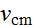of the Center of mass of the wheel.

Is theof two wheels of a bicycle same or different?

Angular speed ω of a rolling wheel is related to the linear speed v of a point on its radius as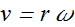Kinetic Energy of Rolling

Rolling is a combination of translation and rotation.

A rolling object has two types of kinetic energies: a rotational kinetic energy due to its rotation about its center of mass and a translational kinetic energy due to translation of its center of mass.

If a wheel of mass M is rotating with an angular speed ω around an axis passing through its center, the kinetic energy due to rotation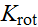is related to its rotation Inertia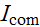as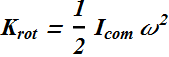Now the wheel also has a linear velocity, so its Kinetic energy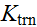due to linear motion will beThe total Kinetic energy K of such a rolling wheel will be sum of these two kinetic energies.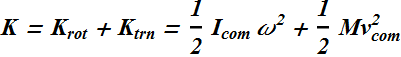Friction and Rolling

How we start rolling of a bicycle?

First we start rotating (click play to start) the wheels by applying torque on the wheel.

If α is the angular acceleration of the rotating wheel of radius R, the tangential accelerations at points T, and P are given as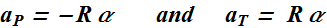For smooth rolling, the velocity at point P should be zero.

How that is possible?

How to find out direction of friction force for any rolling object?

Checkpoint-2: (Rolling)

A circular wheel and a rectangular block were let to go down (Click start) from the top (height h) of a frictionless incline. Both the objects are of same material and have same mass m. Which one will arrive first to the ground?

Rolling down a Ramp

Consider a wheel of mass M and radius R rolling smoothly down a ramp at an angle θ, along x axis.

What will be the direction of friction?

Without friction, the disk will slide down, therefore direction of force of friction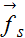will be up (select with friction)

As per Newton's second law, the net force acting on center of mass of the wheel is related to the translational accelerationof its center of mass.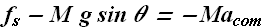Do we know the value of force of friction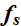?

Remember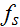is static force of friction (It is not maximum static force of friction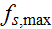). When static force of friction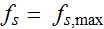, the wheel will start slipping.

How to get the value of?

Since there is rotational motion therefore there should be net torque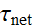acting on the body.

Three forces are acting on the body, static friction,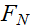and. Torque due toand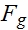is zero (these forces are passing through the axis of rotation). Therefore net torque is only due to force of friction.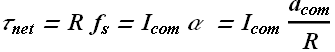Negative sign is the sign of position vector as R will points down ward from center of mass position.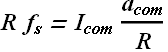By substituting the value ofin first equation we get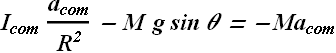By solving above equation we get value of.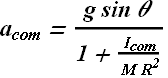The Yo-Yo

Instead of rolling down a ramp at angle θ with the horizontal, the yo-yo rolls down a string at angle θ= 90° with the horizontal.

Instead of rolling on its outer surface at radius R, the yo-yo rolls on an axle of radius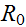.

Instead of being slowed by frictional force, the yo-yo is slowed by the tension force T on it from the string.

Therefore rolling accelerationof the Yo-Yo is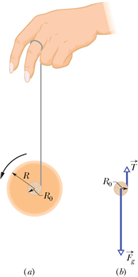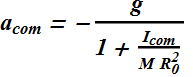Quiz- 2
A hollow cylinder of mass M and radius R rolls down an inclined plane.  A block of mass M slides down an identical inclined plane.  Complete the following statement: If both objects are released at the same time, (Friction is very much present but loss of energy due to friction is negligible)

the cylinder will reach the bottom first.

the block will reach the bottom first.

the block will reach the bottom with the greater kinetic energy.

the cylinder will reach the bottom with the greater kinetic energy.

both the block and the cylinder will reach the bottom at the same time.